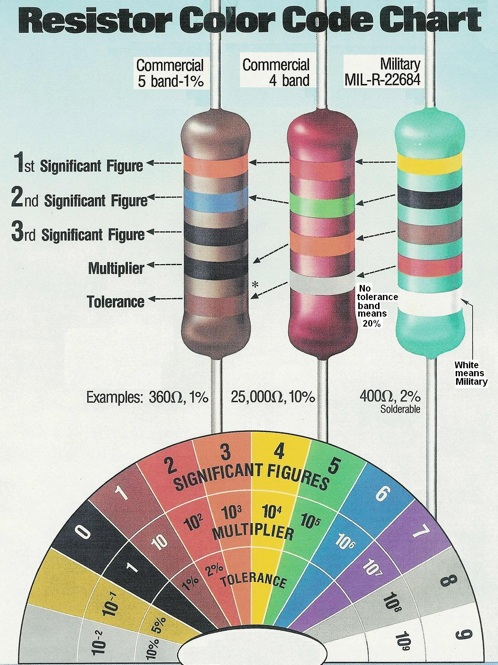Makers, let’s say you could only have 4-5 pages of important charts, formulas and references – what would they be? Here are a few to get you started…

• Resistor color code chart
• Capacitor code chart
• Key to schematic symbols
• Simple circuits to build
• Drill size conversions
• Screw measuring card
• Formulas to calculate areas and volumes
• Common fraction to decimal conversions
• Simple origami (cup, paper airplane)

What are some other handy ones? Diagrams, conversion tables? Post in the comments!

Pictured here, spiffy resistor code chart (along with others here)…

Tagged